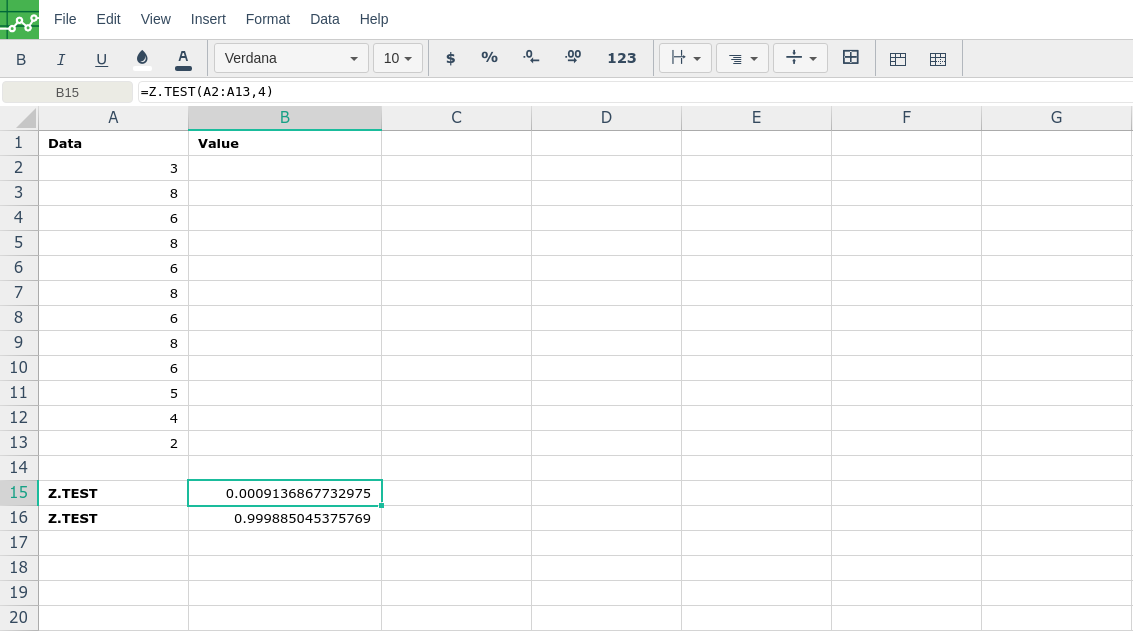# Z.TEST

### Definition

Returns the one-tailed P-value of a z-test.

For a given hypothesized population mean, x, Z.TEST returns the probability that the sample mean would be greater than the average of observations in the data set (array) — that is, the observed sample mean.

To see how Z.TEST can be used in a formula to compute a two-tailed probability value, see the Remarks section below.

### Syntax

Z.TEST(array,x,[sigma])

The Z.TEST function syntax has the following arguments:

• Array     Required. The array or range of data against which to test x.

• x     Required. The value to test.

• Sigma     Optional. The population (known) standard deviation. If omitted, the sample standard deviation is used.

### Notes

• If array is empty, Z.TEST returns the #N/A error value.

• Z.TEST is calculated as follows when sigma is not omitted:

Z.TEST(array,x,sigma) = 1- Norm.S.Dist((Average(array)- x) / (sigma/√n),TRUE)

or when sigma is omitted:

Z.TEST(array,x) = 1- Norm.S.Dist((Average(array)- x) / (STDEV(array)/√n),TRUE)

where x is the sample mean AVERAGE(array), and n is COUNT(array).

• Z.TEST represents the probability that the sample mean would be greater than the observed value AVERAGE(array), when the underlying population mean is μ0. From the symmetry of the Normal distribution, if AVERAGE(array) < x, Z.TEST will return a value greater than 0.5.

• The following formula can be used to calculate the two-tailed probability that the sample mean would be further from x (in either direction) than AVERAGE(array), when the underlying population mean is x:

=2 * MIN(Z.TEST(array,x,sigma), 1 - Z.TEST(array,x,sigma)).

###Error.

User does not have sufficient privileges to access this Content